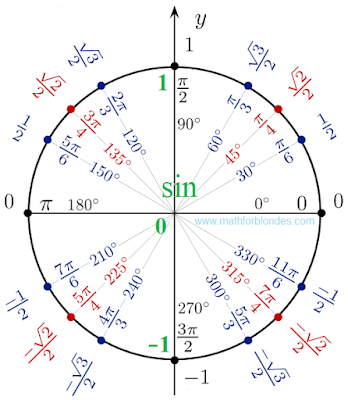## 11.11.2015

### Unit circle

Mathematicians consider themselves clever and all the rest. But not all of us are as smart as mathematics. The unit circle mathematics invented for themselves. For those who are just beginning to study mathematics, I suggest a simpler version - separately cosines and sines separately.

If we remove from the unit circle all that relates to the sine, we get the unit circle cosines.Unit circle cosines

If we remove from the unit circle everything about cosines, we get a unit circle sinuses.Unit circle sinuses

Now, you will not confuse the values of sines and cosines.

## 11.10.2015

### Degrees, minutes, seconds

I was write: "Help me to understand. It is necessary to determine how many degrees, minutes, and seconds contains 4563 seconds corner. Only by actions, and I completely confused."

No harly-barly! We sit quietly and remember that one minute contains 60 seconds, in one degree contains 60 minutes. Now we pimenyaem our knowledge into practice. Transform seconds in a minutes.

4563 : 60 = 76.05 minutes

Determine how many seconds we leave intact:

4563 - 60 * 76 = 4563 - 4560 = 3 seconds

Now 76 minutes translate into degrees, still sadly dividing by 60:

76 : 60 = 1.26667 degrees

We calculate how many minutes do not fit into the clothes degrees:

76 - 60 * 1 = 76 - 60 = 16 minutes

Now our suffering ended and we in good conscience can write the final result:

4563 seconds = 1 degree 16 minutes 3 seconds

Checking? We translate everything back to the second:

1 * 60 * 60 + 16 * 60 + 3 = 3600 + 960 + 3 = 4563

As the math, the conversion is correct.